#Function Repository Resource:

# CanonicalKnuthBendixCompletion

Compute the canonical Knuth-Bendix completion for a given multiway system

Contributed by: Jonathan Gorard
 ResourceFunction["CanonicalKnuthBendixCompletion"][rules] generates a list of canonical (i.e. initial condition-independent) Knuth-Bendix completion rules for the multiway system with the specified rules. ResourceFunction["CanonicalKnuthBendixCompletion"][rules,n] generates a list of canonical (i.e. initial condition-independent) Knuth-Bendix completion rules for the multiway system with the specified rules after n steps.

## Details and Options

Rules can be specified in the following ways:
 {"lhs1"->"rhs1",…} string substitution system {{l11,l12,…}->{r11,r12,..},…} list substitution system WolframModel[rules] Wolfram Model system CellularAutomaton[rules] cellular automaton system "type"→rules system of the specified type
Supported rule types include:
 "StringSubstitutionSystem" rules given as replacements on strings "ListSubstitutionSystem" rules given as replacements on lists "CellularAutomaton" rules given as a list of CellularAutomaton rule specifications "WolframModel" rules given as replacements on hypergraphs
ResourceFunction["CanonicalKnuthBendixCompletion"] accepts both indiviudal rules and lists of rules.
Knuth-Bendix completion transforms an arbitrary rewrite system into a confluent (causal invariant) rewrite system by resolving critical pairs.
In the context of the multiway systems, critical (branch) pairs indicate bifurcations or ambiguities in the multiway evolution.
ResourceFunction["CanonicalKnuthBendixCompletion"] gives the minimal set of rules required to force all unresolved canonical branch pairs to converge.

## Examples

### Basic Examples (2)

Generate the list of all canonical Knuth-Bendix completion rules for a string substitution system:

 In:=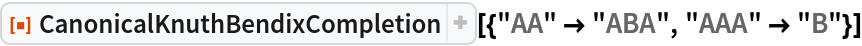Out=Generate the list of canonical Knuth-Bendix completion rules necessary to force confluence after 2 steps:

 In:=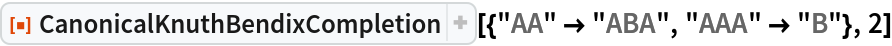Out=CanonicalKnuthBendixCompletion can handle Wolfram models and other system types:

 In:=Out=In:=Out=Provide a cellular automaton as input:

 In:=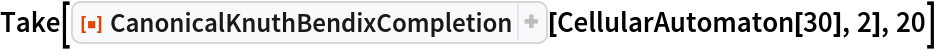Out=### Scope (4)

#### System Types (4)

CanonicalKnuthBendixCompletion supports both string and list substitution systems:

 In:=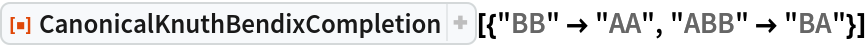Out=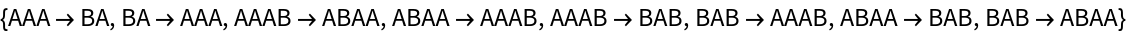Use substitutions on lists:

 In:=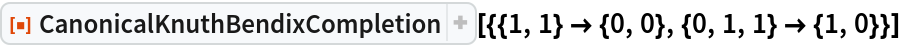Out=CanonicalKnuthBendixCompletion also supports multiway generalizations of cellular automata:

 In:=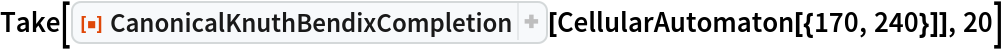Out=Generate a Knuth-Bendix completion for left- and right-shift cellular automaton rules after 5 steps:

 In:=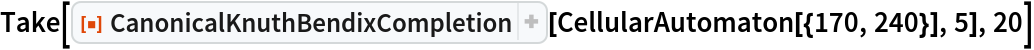Out=Determine that the rule 30 cellular automaton is not total causal invariant:

 In:=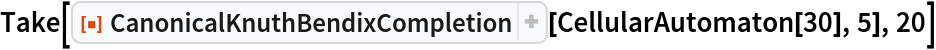Out=CanonicalKnuthBendixCompletion also supports multiway generalizations of Wolfram Models:

 In:=Out=Determine that this Wolfram model rule is not total causal invariant:

 In:=Out=### Applications (2)

#### Causal Invariant Rules (2)

Prove that the Xor and And functions are total causal invariant (due to associativity):

 In:=Out=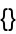In:=Out=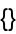On the other hand, the Nand function is not associative, so it is not total causal invariant:

 In:=Out=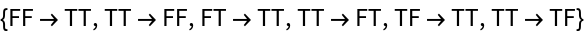### Properties and Relations (1)

CanonicalKnuthBendixCompletion returns an empty list of canonical Knuth-Bendix completion rules if and only if the rule is total causal invariant:

 In:=Out=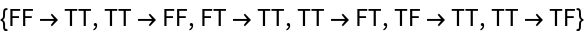In:=Out=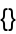## Version History

• 1.0.0 – 13 April 2020